# Problem 52070. Compute the effective conductivity of a heterogeneous aquifer

Slow flow through soil or another porous medium follows Darcy’s law, which relates the flow of water Q to the gradient in piezometric head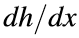bywhere K is the conductivity of the soil and A is the flow area (i.e., the aquifer thickness multiplied by the aquifer width). The flow Q (and the specific discharge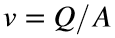) is analogous to current in an electrical circuit, and the change in head is analogous to the voltage drop.
Some aquifers, or underground water-bearing formations, consist of multiple soil units in series or parallel, as shown below. The analysis of these aquifers is often simplified by computing the effective conductivity—that is, treating the aquifer as homogeneous with the single value of K set such that the aquifer produces the same flow under the same total change in head.
For example, suppose the soil units have equal size in the two aquifers shown below and flow in both cases is from left to right. If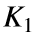is 2 m/d (meters/day) and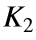is 6 m/d, then the effective conductivity is 4 m/d in the parallel case and 3 m/d in the series case.
Write a function that computes the effective conductivity of an aquifer whose conductivity (in m/d) is specified as a matrix. Flow is always left to right, and heterogeneous cases involve either units in series or units in parallel. In the series case, the conductivity will be constant in each column, and in the parallel case, the conductivity will be constant in each row. The relative size of each unit is indicated by the number of rows or columns.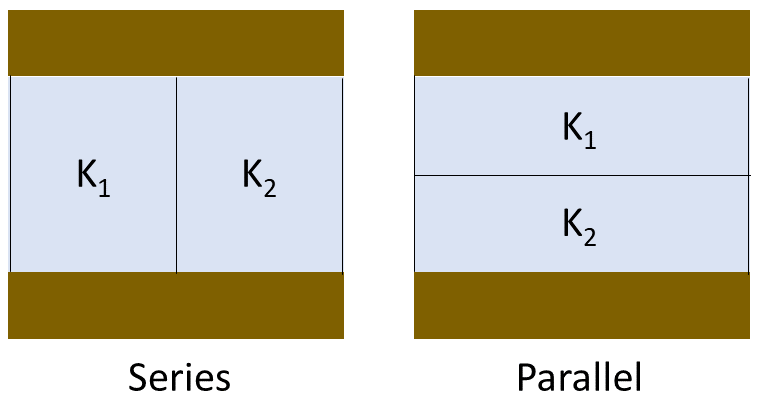### Solution Stats

60.0% Correct | 40.0% Incorrect
Last Solution submitted on Aug 24, 2023

### Community Treasure Hunt

Find the treasures in MATLAB Central and discover how the community can help you!

Start Hunting!